## Examples Of Event Extraction And RemovalIn many cases only significant events, such as bumps or other transients in a signal are of relevance. The objective is to be able to isolate these events in a meaningful manner so that they may be automatically recognised and either removed or extracted for analysis in a structured way.

There are two principle objectives initially: one is to be able to recognise an event and the other is to be able to mark it in some way so that subsequent software is able to operate on the actual event. We must also note that an event has a start and an end; the criterion we use to recognise the start may not necessarily be the same criterion we use to recognise the end. Searches for the start and end points are carried out on a Reference Signal. How the reference signal is formed is discussed in detail later, it includes the original signal, various running statistical measures such as the dynamic RMS, differentiation for slope detection, integration and so on. In many cases the start criterion will be some check on the level achieved by the reference signal. By the time any check level has been detected then it is almost certain that the event started earlier! That is, a pre trigger capability is essential.

### Start and End Point Detection

The types of amplitude level detection currently supported for the Start Point of the event are illustrated in Figure 1.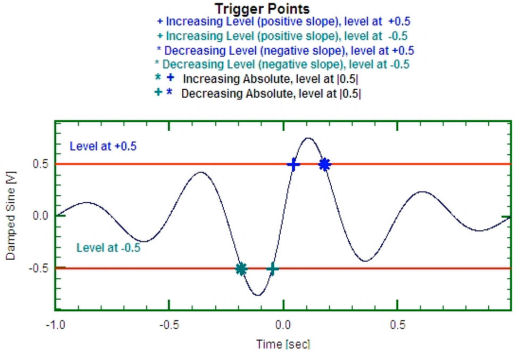Figure 1: Amplitude Level Detection

Specifying the End Point has all of the same choices as for the Start Point but most importantly with two additional choices:

• post trigger duration;
• dB below Peak.

The duration criterion is very straightforward. In Figure 2. the reference signal was selected as a time history. The start of an event has been detected using a simple increasing level set at 20m/sec2. The post trigger duration was set at 1.5 seconds and the pre trigger duration was set at 0.5 seconds, giving a total duration of 2 seconds.Figure 2: Event Detection with pre and post trigger

Using an end of event determined by the dB below peak method does require more explanation. This type of end of event criterion is clearly not appropriate with a time history as the reference signal, not least because a time history is negative as well as positive and it is not possible the take dB’s of negative values! So in the following example the dynamic rms of the original time history has been calculated as the reference signal. (Note. The dynamic rms is the rms of the signal with the mean value removed, this is the same as the standard deviation). As the software will look for the maximum level of the reference signal following the current event start point, then clearly an extra parameter is required to limit the maximum search duration. The search range is defined as the region from the event start point to the start point plus the use defined maximum search length, or until the end of the signal if there is insufficient data. The software finds the maximum value of the reference signal in this search range.Figure 3: Searching using "dB below peak"

Once the maximum value in the search range is found then it is straightforward to compute the amplitude of the target end point. The software then searches from the location of the maximum value until it either finds the level or until it reaches the end of the search range. The behavior is illustrated in Figure 3 above. In this case the start trigger level was set at 10dB, the end detect as 30dB below the peak level and the maximum search duration as 2 seconds.

 Event Type Operation Increasing Level The trigger point occurs when the signal crosses the specified level with a positive slope. That is, it crosses from a less positive value to a more positive value. The specified level may of course be either positive or negative. Decreasing Level The trigger point occurs when the signal crosses the specified level with a negative slope, that is it crosses from a more positive value to a less positive value. The specified level may of course be either positive or negative. Increasing Absolute If the specified level is say A then there are two levels set, one at +A and the other at –A, forming a corridor. The trigger point here occurs when the signal exits out of the corridor. If at the start the signed is already outside the corridor, then the signal has to re enter the corridor before an exit is determinable. Decreasing Absolute If the specified level is say A then there are two levels set, one at +A and the other at –A, forming a corridor. The trigger point here occurs when the signal enters into the corridor.

### Calculation of the Reference Signal (Reference Setup)

The Extract Event module uses one of the signals in the dataset as the basis for the reference signal. The simplest situation is when the signal is used directly, this is referred to as raw data. Time varying versions of the reference signal include:

• Raw data
• Mean level
• Dynamic RMS (standard deviation – removes effect of mean level)
• RMS
• Skewness
• Kurtosis
• M5 (fifth moment)
• M6 (sixth moment)

Any one of the above time varying variants may be selected as part of the Reference Signal. It may then be raised to a power and combined with another of the above variants, also raised to a different power. If required, the result may be integrated or differentiated to form the Reference Signal. The method of combining is the usual arithmetic operators +, -, *, and / together with the special operator NULL. If the NULL operator is used then the second Variant is ignored.

It we denote the selected variant as Vi and another variant as Vk then the possibilities are shown below.The final option for the calculated reference signal is to normalise. This operation gives the reference signal a unit standard deviation and, optionally, an overall zero mean. This aids selection of the trigger level by making the signal non dimensional, and the trigger level may be assessed in terms of number of Standard Deviations of the reference signal. Normalising does not affect the ‘dB below the peak’ criterion as this is a relative measure. Examples of normalising different types of reference signals are given below.

In many cases using the amplitude alone is sufficient. Squaring (raising to power 2) eliminates all negatives, which is useful on occasion. The kurtosis and M6 functions are defined as being normalised by the dynamic rms (standard deviation) so multiplying by the dynamic rms raised to the appropriate power removes the normalisation.

After the raw data itself, the most frequently used classifier is the dynamic rms as this represents the variation of the total power in the signal with time. It is also one of the most convenient reference signals to use with the End of Event detector number of dB’s below the peak.
When dealing with transients then consider using the Kurtosis and M6 functions.

### Illustration of Slope Detection (Differentiated Reference)

Slope detection works on the derivative of the reference signal. It is very useful when there is a sharp change of slope. The slope detector is often very useful to distinguish between different types of events. The signal below was taken as part of a study on “passby events”. Generally these events last several seconds. On occasions during the tests the vehicle hit a bump which caused a sharp impulsive type of event. As the test parameter classifying the events was the peak acceleration, the bumps could noticeably affect the results. Some bumps were clearly higher than the levels reached by the passby events so these could be readily identified and removed using the amplitude level criteria. However many were of the same order of magnitude as the passby events. In this situation using the derivative of the signal as the reference readily identifies the two different types of event.

Figure 4 shows a section of the captured signal. Most of the passby events take around 10 to 15 seconds but at around the 610 second mark notice an apparently isolated spike of the same general peak level as the passby events.

Expanding the section around the six hundred and eleven seconds point (Figure 5) shows an impulse response due to the bump which lasts perhaps a quarter of a second.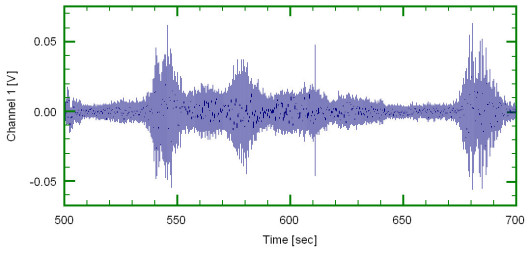Figure 4: Passby events with spikeFigure 5: Expanded section of signal

Because the bump amplitude is of the same order of magnitude as the passby events then using an amplitude discriminator on the time history is clearly not viable.

If, as shown in Figure 6, the derivative of the signal is used then a clear distinction emerges. In figure 6 the top graph is the original time history and the lower one is the slope (first derivative) of the amplitude curve.

After applying the relative removal method the result is shown in Figure 7, again compared with the original time history.Figure 6: Differentiating the signal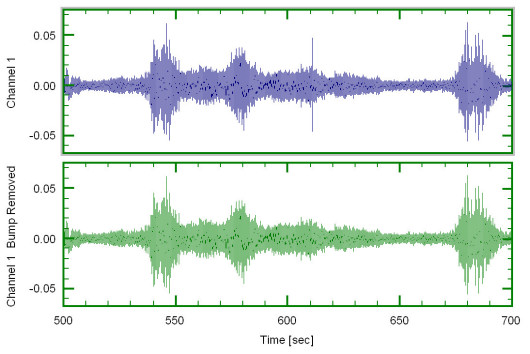Figure 7: Spike removed

However a word of caution, noisy low amplitude signals will often have significant slope changes leading to erroneous event detection so care is advisable.

### Integration Example

Where differentiation picks out rapid changes, integration smooths short term trends and reveals any slow varying events. It we applied integration directly we may not obtain what we thought! Using the same data as above then just integrating gives the effect shown in Figure 8.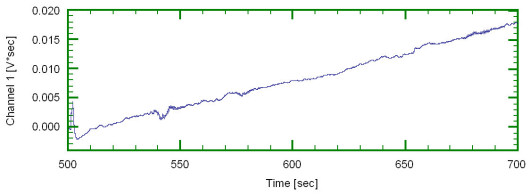Figure 8: Integrating the signal

The reason for the increasing slope is that there is an underlying positive mean value which when integrated causes the upward effect. We may remove this effect by forming the reference signal from:

reference = ? (RawData – MeanTrend) dt

where all the integrations are the rolling type over a short period.

The reference signal then looks like the example in Figure 9.Figure 9: Rolling integrationFigure 10: Comparison with original signal

Expanding the first part of the signal and comparing it to the original signal shows (Figure 10) the slow term variations at around the 500 to 503 second mark.

In the time history the amplitude of the slow varying disturbance is lower than the main passby events. Clearly using simple amplitude discrimination would not prove useful. However the integration of the running mean adjusted signal may be used as a suitable reference. In the study in hand the slow varying effects were not large enough to create concern. However if they had been then we may identify them and thus remove them automatically.

### Illustration of Normalising

Again using the same example data, the figure below shows three different reference signals constructed from the original signal. The top reference signal is the dynamic rms as a function of time. The centre reference signal is the differentiation of the original signal and the bottom reference signal is the time varying mean adjusted integration.

Each reference signal shows (Figure 11) the expected characteristics but note the very different Y axis scales.Figure 11: Three different reference signals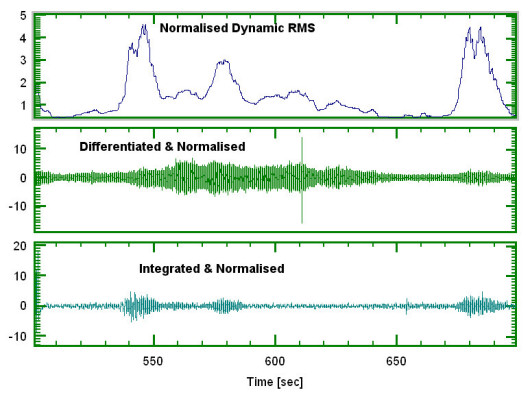Figure 12: Normalised reference signals

The second set of three reference signals in figure 12 is the same shape as the above graphs but now the references have all been normalised and the Y axis scales are much more similar. In nearly all cases a trigger level of somewhere between two and ten would be used. As noted earlier normalising does not affect the ‘dB below peak’ end point detection scheme.

### Marking Events

This is very simple within DATS as the event recognition software makes use of the Named Element facility to store the location of the beginning of each event, the end of each event and the total number of events as illustrated in Figure 13.Figure 13: DATS “Named Element” view

The beginning and end locations are given both in terms of physical units of the X axis (the independent variable) and as the point number in the signal. The Beginning Point includes any pre-trigger interval so it is not necessarily the same as the Event Start Point. We can see for example that Event 1, including the pre-trigger period, began at 525.30625037709251 seconds and ended at 546.00703193555819 seconds, corresponding to point numbers 64785 and 117779 respectively. The marking scheme has the capability to recognise up to 99999 separate events in a single signal. Note that the Named Elements are the temporary type (they start with the # symbol) so by design they are not propagated.

As well as storing the locations in the Named Elements in the original signal the event recognition software will optionally store the ‘mask’ signal and the actual reference signal used in the original Dataset. This allows visual checking subsequently as illustrated in Figure 14.

It also allows another method of extracting the events by simply multiplying the data by the mask signal. If tapering is selected this will also be included in the mask signal as illustrated in Figure 15 where a rather large taper has been used for ease of visualising.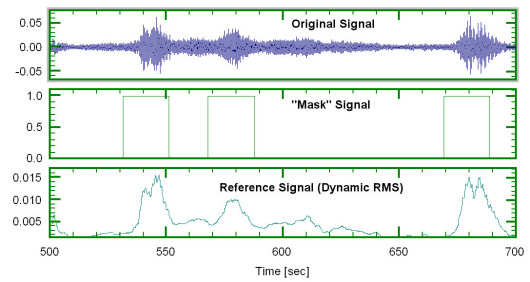Figure 14: Illustration of “mask” signalFigure 15: “Mask” with 40% taper

### Style of Extracted Events

Once a set of events has been identified then the extraction or removal may be applied either to just the original signal or, more usually, to all signals in the dataset. With extraction there are three options, for removal only the first two options apply:

1. Extract/Remove and maintain relative position;
2. Extract/Remove and concatenate events;
3. Extract each event to a separate dataset.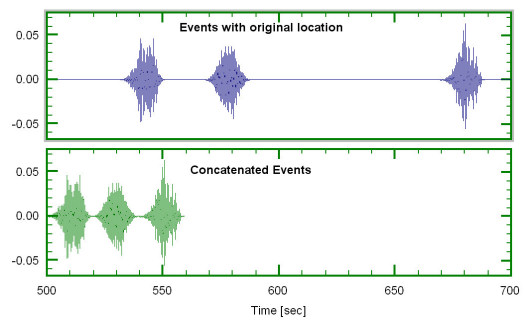Figure 16: Two types of event extractionFigure 17: Events extracted into separate signals

The examples (Figures 16 and 17) show the three types of event extraction. For clarity tapering has been applied so that individual events may still be recognised when concatenated.

The following two tabs change content below.#### Dr Colin Mercer

Chief Signal Processing Analyst (Retired) at Prosig
Dr Colin Mercer was formerly at the Institute of Sound and Vibration Research (ISVR), University of Southampton where he founded the Data Analysis Centre. He then went on to found Prosig in 1977. Colin retired as Chief Signal Processing Analyst at Prosig in December 2016. He is a Chartered Engineer and a Fellow of the British Computer Society.## One thought on “Examples Of Event Extraction And Removal”

1.Andrey Krishkevich

Great article! Very precise and sufficiently pedagogical. Thank you!

P.S. You might also talk about wavelet transform for detecting discontinuities on several differential levels.

This site uses Akismet to reduce spam. Learn how your comment data is processed.

1. We welcome any feedback, questions or commentsCLOSE
Optimization WordPress Plugins & Solutions by W3 EDGE